Courses

# Signal Flow Graphs

## 10 Questions MCQ Test Topicwise Question Bank for Electronics Engineering | Signal Flow Graphs

Description
This mock test of Signal Flow Graphs for Electrical Engineering (EE) helps you for every Electrical Engineering (EE) entrance exam. This contains 10 Multiple Choice Questions for Electrical Engineering (EE) Signal Flow Graphs (mcq) to study with solutions a complete question bank. The solved questions answers in this Signal Flow Graphs quiz give you a good mix of easy questions and tough questions. Electrical Engineering (EE) students definitely take this Signal Flow Graphs exercise for a better result in the exam. You can find other Signal Flow Graphs extra questions, long questions & short questions for Electrical Engineering (EE) on EduRev as well by searching above.
QUESTION: 1

### Mason's rule is applied to

Solution:

Mason's rule or Mason’s gain formula is applied to signal flow graph for finding the transfer function.

QUESTION: 2

### Assertion (A): Signal flow graph is applicable to the linear systems. Reason (R): Signal flow graph method of finding the transfer function of a system is very simple and does not require any reduction technique.

Solution:

Signal flow graph is applicable to the linear systems because output is proportional to gain of the system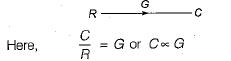Reason (R) is also true but is not a correct explanation of assertion (A)

QUESTION: 3

### Consider the following three cases of signal flow graph and their corresponding transfer functions: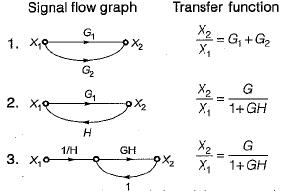Q. Which of the above relations is/are correctly matched?

Solution: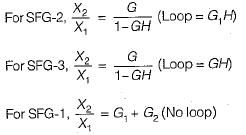Hence, only 1 is correctly matched.

QUESTION: 4

The signal flow graph shown below has M number of forward path and N number of individual loops.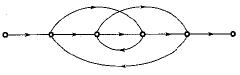Q. ​What are the values of M and N ?

Solution:
QUESTION: 5

The signal flow graph for a certain feedback control system is given below.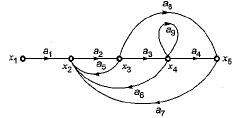Now, consider the following set of equations for the nodes:

1.  x2= a1x1+a5x3+a6x4+a7x5
2. x3=a2x
3. x4=a3x
4. x5=a4x4+a8x3

Q. Which of the above equations are correct?

Solution:

Correct Answer :- b

Explanation : x2 = a1x1 + a5x3 + a6x4 + a7x5

x3 = a2x2

x4  a3x3 + a9x5

x5 = a4x4 + a8x3

QUESTION: 6

The gain C(s)/R(s) of the signal flow graph shown below is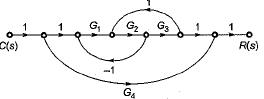Solution:

Gain of forward paths are:
P1 = G1G2G3 and P2 = G4
Here, Δ1 = 1
and Δ2 = (1 + G1G2— G2G3)
Individual loops are:
L1 = -G1G2
and L2 = G2G3
Non-touching loop = Nil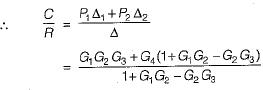QUESTION: 7

The number of forward paths and the number of non-touching loop pairs for the signal flow graph shown in the figure below are respectively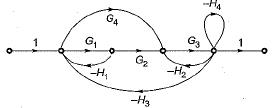Solution:

Forward paths are
P1 = G1G2G3 and P2 = G3G4
Gain product of non-touching loop pairs are:
L1 L2 = G1H1H4
and L1L3 = G1G3H1H2
Thus, there are two forward paths and two non touching loop pairs

QUESTION: 8

The signal flow graph of the RLC circuit of figure (i) is shown in figure (ii)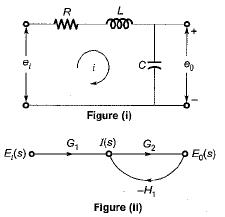The values of G1 and H1 are respectively

Solution:

Converting the given circuit in s-domain and writing the equations, we get: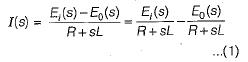Also, from given SFG, we have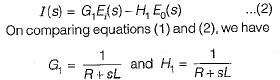QUESTION: 9

The closed loop transfer function CIR for the signal graph shown below is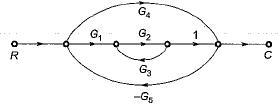Solution:

Number of forward paths are two,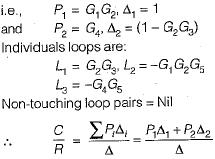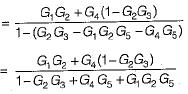QUESTION: 10

Consider the following statements with regards to the signal flow graph shown below.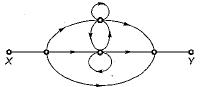1. The number of forward paths are 2.
2. The number of forward paths are  3.
3. The number of loops are 3.
4. The number of loops are 5,
5. The number of non-touching loop pairs is 1.
6. The number of non-touching loop pairs is nil.

Q. Which of these statements are correct?

Solution: